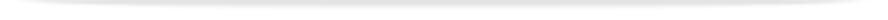units

MAT2731

Faculty of Engineeringprint version

This unit entry is for students who completed this unit in 2016 only. For students planning to study the unit, please refer to the unit indexes in the the current edition of the Handbook. If you have any queries contact the managing faculty for your course or area of study.

# MAT2731 - Multivariate analysis

## 6 points, SCA Band 2, 0.125 EFTSL

Refer to the specific census and withdrawal dates for the semester(s) in which this unit is offered.

Faculty

Engineering

Coordinator(s)

Offered

Gippsland

• First semester 2016 (Day)
• First semester 2016 (Off-campus)

### Synopsis

Multivariable functions, partial differentiation and optimization. Vector analysis with physical applications. Integration in three dimensions: along curves, over surfaces and throughout regions of space. Identities including Gauss's divergence theorem and Stokes' theorem. The continuity, momentum and energy equations for fluid flow, expressed in 3D vector form. Mass transport (diffusion and advection), diffusion across a liquid/gas interface and light availability (Lambert-Beer model). Random variables, their probability distributions and expected values as summary measures. The Poisson, normal, exponential distributions and distributions useful in the analysis of extremes. Point and interval estimation of model parameters. Simple linear regression and correlation.

### Outcomes

On completion of this unit, a student is expected to have developed: an enhanced appreciation of the analytic approach to the solution of engineering science problems; mathematical manipulative skills appropriate to the analysis tools; and an appreciation of the benefits and limitations of mathematical analysis and of the need to interpret a mathematical solution in the context of the engineering problem. The student is also expected to have developed: statistical skills for the analysis of data, and the ability to calculate confidence intervals for means.

### Assessment

Three assignments (10%, 15%, 15%): 40%
Examination (3 hours): 60%

3 hours lectures, 2 hours tutorials/ PC laboratory classes and 7 hours of private study per week